Refer to our Texas Go Math Grade 1 Answer Key Pdf to score good marks in the exams. Test yourself by practicing the problems from Texas Go Math Grade 1 Lesson 4.4 Answer Key Add Zero.

Essential Question
What happens when you add 0 to a number?
Explanation:
Identity Law of Addition states that any number added to 0 is equal to itself.
Therefore, you can add any number and get the same sum

Explore
Useto model the problem. Draw theyou use.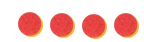Explanation:
Scott has 4 marbles.
Jennifer has no marbles.
4 + 0 = 4
4  marbles that they have

For The Teacher

• Read the following problem. Scott has 4 marbles. Jennifer has no marbles. How many marbles do they have?Explanation:
Scott has 4 marbles.
Jennifer has no marbles.
4 + 0 = 4
4  marbles that they have

Math Talk
Mathematical Processes
Explanation:
Identity Law of Addition states that any number added to 0 is equal to itself.
Therefore, you can add any number and get the same sum

Model and Draw

What happens when zero is added to a number?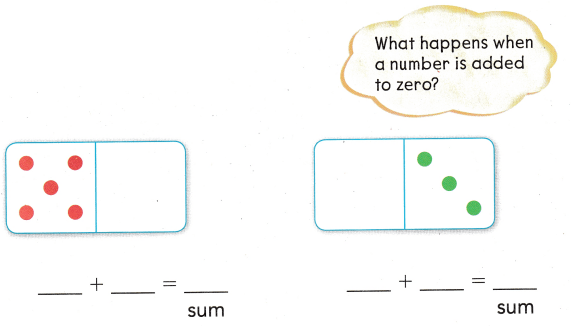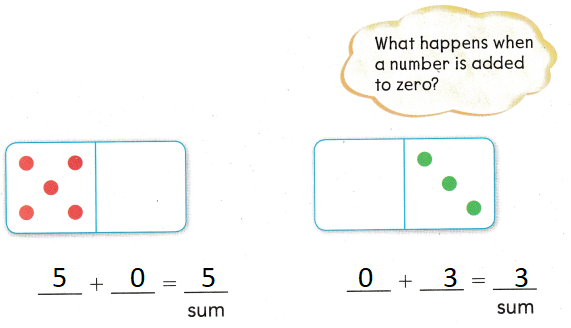Explanation:
When a 0 is added to a number the answer will be itself

Share and Show

Use the picture to write each part. Write the sum

Question 1.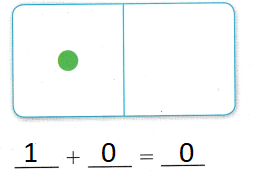1 + 0 = 0
Explanation:
When a 0 is added to a number the answer will be itself

Question 2.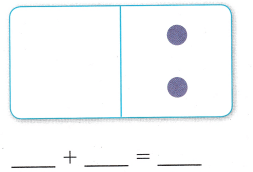Explanation:
When a 0 is added to a number the answer will be itself

Question 3.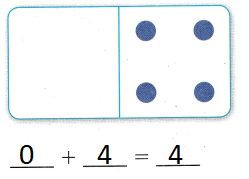Explanation:
When a 0 is added to a number the answer will be itself

Question 4.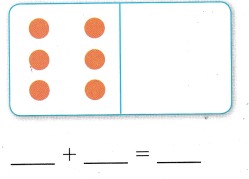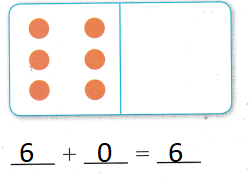Explanation:
When a 0 is added to a number the answer will be itself

Problem Solving

Draw circles to show the numbers. Write the sum.

Question 5.

3 + 0 = ___
3 + 0 = 3
Explanation:
When a 0 is added to a number the answer will be itself

Question 6.

1 + 4 = ___
1 + 4 = 4
Explanation:
The sum of 1 and 4 is 5

Question 7.

4 + 0 = ___
4 + 0 = 4
Explanation:
When a 0 is added to a number the answer will be itself

Write or draw to solve.

Question 8.
There are 3 dogs on the path. No more dogs join them. How many dogs are on the path?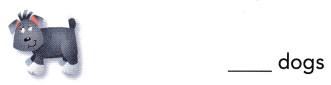Explanation:
When a 0 is added to a number the answer will be itself

Question 9.
H.O.T. Multi-Step Maya has 7 books. Eli has no books. Then Maya gives 7 books to Eli. How many books does Maya have?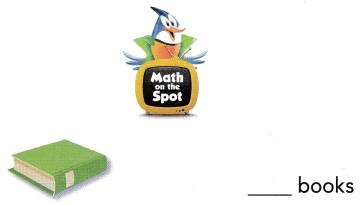Explanation:
0 books that Maya have
7 + 0 = 7

Question 10.
There are 5 red birds. There are 3 green birds. How many birds are blue?Explanation:
3 + 5 = 8
8 + 0 = 8
0 birds are blue.

Question 11.
One chicken has 0 eggs in her nest. Another chicken has 7 eggs in her nest. How many eggs do the chickens have?
(A) 7
(B) 6
(C) 9
Explanation:
0 + 7 = 7
7 eggs that the chickens have

Question 2.
Molly has 4 seahorses in her fish tank. She has 0 fish. Which number sentence shows the number of fish and seahorses she has?(A) 4 + 1 = 5
(B) 4 + 0 = 4
(C) 4 + 2 = 6
Explanation:
4 + 0 = 4
Is the number sentence that shows the number of fish and seahorses she has

Question 13.
Analyze Jody has 6 rocks in her fish tank. 6 rocks are red. How many blue rocks does Jody have in her fish tank?
(A) 0
(B) 6
(C) 8
Explanation:
0 + 6 = 6
0 blue rocks that Jody have in her fish tank

Question 14.
Texas Test Prep What is the sum of 0 + 6?
(A) 0
(B) 7
(C) 6
Explanation:
the sum of 0 and 7 is 7

Take Home Activity

• Write the numbers 0 to 9 on small squares of paper. Shuffle and place the cards facedown. Have your child turn the top card, and add zero to that number. Say the sum. Repeat the activity for each card.
Explanation:
1 + 0 = 1
2 + 0 = 2
3 + 0 = 3
4 + 0 = 4
5 + 0 = 5 as on

### Texas Go Math Grade 1 Lesson 4.4 Homework and Practice Answer Key

Write or draw to solve.

Question 1.

6 + 0 = ___
6 + 0 = 6
Explanation:
When a 0 is added to a number the answer will be itself

Question 2.

1 + 5 = ___
1 + 5 = 6
Explanation:
The sum of 1 and 5 is 6

Question 3.

3 + 0 = ___
3 + 0 = 3
Explanation:
When a 0 is added to a number the answer will be itself

Problem Solving

Question 4.
There are 5 children playing a game. No more children join them. How many children are playing the game?
___ children
5 children
Explanation:
5 + 0 = 5
5 children are playing the game

Question 5.
Multi-Step Taylor draws 8 pictures. Dylan draws no pictures. How many pictures do they draw?
____ pictures
Then Taylor gives 8 pictures to Dylan. How many pictures does Taylor have?
____ pictures
8 + 0 = 8
Taylor has 8
0 + 8 = 8
Dylan has 8
Explanation:
Taylor draws 8 pictures. Dylan draws no pictures.
In this case Taylor has 8
Then Taylor gives 8 pictures to Dylan.
In this case Dylan has 8

Texas Test Prep

Lesson Check

Question 6.
One bike rack has 0 bikes. Another bike rack has 9 bikes. How many bikes are in bike racks?
(A) 9
(B) 0
(C) 10
Explanation:
0 + 9 = 9
9 bikes are in bike racks

Question 7.
Leah has 3 red hats. She has 0 blue hats. Which number sentence shows the number of hats she has?
(A) 3 + 2 = 5
(B) 3 + 0 = 3
(C) 3 + 1 = 4
Explanation:
3 + 0 = 3
Is the number sentence that  shows the number of hats she has

Question 8.
What is the sum of 0 + 7?
(A) 0
(B) 9
(C) 7
Explanation:
0 + 7 = 7
When a 0 is added to a number the answer will be itself

Question 9.
Multi-Step Cooper has 5 soccer balls and 0 basketballs. Liam has 2 soccer balls and basketballs. How many balls do they have?(A) 7
(B) 11
(C) 9